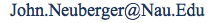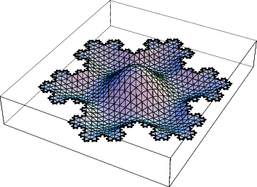## John M. Neuberger

### Associate Professor of Mathematics Department of Mathematics and Statistics College of Engineering, Forestry, and Natural Sciences Northern Arizona UniversityJune 2012 Variational and Topological Methods Conference

 Fall 2012 Course Schedule: MAT136 - Calculus I MAT431, Advanced Calculus. Vita. Research Statement. Papers.

Some Current Research.Bifurcation DiagraphDecorated Cayley graph for Z5Solution on the Dodecahedron with Anomalous Invariant Symmetry related to identification to the Petersen graphA CCN solution on the Truncated Icosahedron, a MI 2 minimal energy solution which changes sign exactly once

Some Other Research.Bif Diag, sym/asym sol: u''+s*u +4(x-.5)^2u^3=0 on (0,1) u(0)=0=u(1)Triple Junction on Disk6th eigenfunction of the Laplacian on a Snowflake. Bifurcation Diagram for Triple Well System Equivalence Classes of Solutions near primary bifurcation point off of trivial (local max) branch for Triple Well System Neumann BC e-fncts on Disk 13+31 tertiary branch non-odd 12+21 branch possible G-L vortex? Plotting $u:C\to C$ as vector field